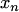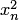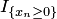# History Of Game-theoretic Probability

Already in the 1654 correspondence between Pascal and Fermat one can discern the appearence of game-theoretic (Pascal's solution to the problem of points) and measure-theoretic (Fermat's solution) methods. To solve the problem, Pascal constructs a martingale, and Fermat counts favourable cases. (See, e.g., http://www.probabilityandfinance.com/articles/32.pdf, Section 2.1, for details.)

The sixth of the 23 celebrated problems that Hilbert presented in 1900 was to treat axiomatically, after the model of geometry, those parts of physics in which mathematics already played an outstanding role, especially probability and mechanics. As far as probability is concerned, Richard von Mises's game-theoretic response was first published in 1919, and Kolmogorov's measure-theoretic response in 1931 and 1933 (see measure-theoretic probability). Whereas von Mises defined probability using a principle of the impossibility of a gambling system, Kolmogorov axiomatized probability as a special case of measure.

In 1939 Jean Ville noticed that von Mises's definition was not sufficient to cover the law of the iterated logarithm, and introduced the notion of martingale to rectify it. Whereas von Mises's gambling systems choose a subsequence of trials on which to bet, Ville considered more sophisticated gambling systems which also vary the amount of the bet and the outcome on which to bet. Joseph Doob reviewed Ville's book for Mathematical Reviews, and later translated the notion of martingale into Kolmogorov's measure-theoretic probability and greatly developed the mathematics of martingales.

An advantage of game-theoretic probability is that we do not need to assume that every measurable event is assigned a probability, or that every bounded random variable is assigned an expectation. We assume as few probabilities/expectations as possible for a specific result, such as the strong law of large numbers for bounded observations (where Forecaster only pricesand not, e.g.,or).

Andrei Kolmogorov (in 1963 – 1970) tried to revive von Mises–type game-theoretic probability, creating the algorithmic theory of randomness. Levin and Schnorr extended Kolmogorov's ideas to Ville-type randomness. But these authors did not emphasize the possibility of weakening probabilistic assumptions. This step was made by A. P. Dawid in his prequential statistics (1984), and especially in his prequential principle.

### Bibliography

• A. P. Dawid (1984). Statistical theory: The prequential approach. Journal of the Royal Statistical Society A, 147:278 - 292.
• Richard von Mises (1919). Grundlagen der Wahrscheinlichkeitsrechnung. Mathematische Zeitschrift, 5:52 - 99.
• Jean Ville (1939). Etude critique de la notion de collectif. Gauthier-Villars, Paris.